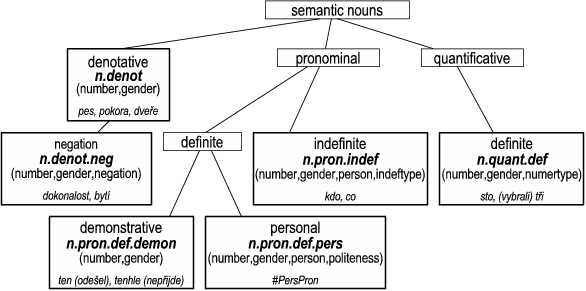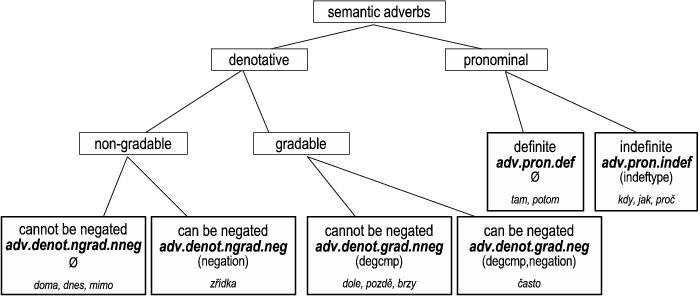### 2.2. Inner structure of the semantic parts of speech

!!! Semantic verbs are not further classified so far.

The information regarding the semantic part-of-speech character of a complex node is encoded in its `sempos` attribute; also the information as to which subgroup the particular semantic noun, adjective or adverb belongs to is included. The possible values of the `sempos` attribute are to be found in Table 5.1, "Values of the `sempos` attribute".

The inner structure of semantic nouns, adjectives and adverbs is to some extent parallel, however, not identical.

The inner structure of the semantic parts of speech is represented with the help of a tree structure (graph) the root of which is the label of the given semantic part of speech. The first level below the label lists the basic subgroups of the class in question (i.e. the denominating, pronominal and quantificational subgroups); further classification follows on the lower levels. Below the label of a subgroup, the value of its `sempos` attribute is given (in boldface). Below the value of the `sempos` attribute, there is a set of grammatemes that are associated with the given subgroup (if there are no grammatemes associated with the given subgroup, it is indicated by the ∅ symbol); below the grammatemes, there are examples of possible t-lemmas.

#### 2.2.1. Inner structure of semantic nouns

The inner structure of semantic nouns is presented in Fig. 5.2.

Figure 5.2. Inner structure of semantic nounsThe subgroup of denominating semantic nouns (`sempos`=`n.denot`; see Section 6.1.1, "Denominating semantic nouns") includes - apart from the traditional nouns - also possessive adjectives represented by the t-lemmas of the corresponding semantic nouns.

Within the denominating semantic nouns, there is a subgroup of nouns (namely nouns ending with -ní / -tí and -ost) for which it is possible to separate the negation from the rest of the lexical content of the word (`sempos`=`n.denot.neg`; see Section 6.1.1.1, "Denominating semantic nouns with which the negation is represented separately").

!!! This is only a temporary solution: in fact, all denominating semantic nouns should be divided into two groups depending on whether they can be negated or not. This would lead to distinguishing two subgroups of denominating semantic nouns, the situation which can be found with denominating semantic adverbs.

Definite pronominal semantic nouns are divided into demonstrative pronouns (`sempos`=`n.pron.def.demon`; see Section 6.1.2, "Definite pronominal semantic nouns: demonstratives"); these are demonstrative pronouns in the position of syntactic nouns (e.g.: ten nepřijde (=lit. this not_comes)); and personal pronouns (`sempos`=`n.pron.def.pers`; see Section 6.1.3, "Definite pronominal semantic nouns: personal pronouns"), these are personal and possessive pronouns including the reflexives (they are all represented by a single t-lemma: `#PersPron`).

The subgroup of indefinite pronominal semantic nouns (`sempos`=`n.pron.indef`; see Section 6.1.4, "Indefinite pronominal semantic nouns") consists of relative, indefinite, interrogative, negative and totalizing pronouns and their possessive counterparts. The subgroup of definite quantificational semantic nouns (`sempos`=`n.quant.def`; see Section 6.1.5, "Definite quantificational semantic nouns") consists of numerals in the positions of syntactic nouns, i.e. cardinal numerals (e.g.: Přišli tři / Vybrali tři z pěti nabízených knih (=lit. Came three / Chose three from (the) five offered books)), as well as fraction numerals (e.g.: třetina (=one_third) represented by tři (=three)).

#### 2.2.2. Inner structure of semantic adjectives

The inner structure of semantic adjectives is presented in Fig. 5.3.

Figure 5.3. Inner structure of semantic adjectivesThe subgroup of denominating semantic adjectives (`sempos`=`adj.denot`; see Section 6.2.1, "Denominating semantic adjectives") includes traditional adjectives (except for the possessive adjectives which belong to the class of semantic nouns), as well as deadjectival adverbs which are represented by the t-lemmas of their adjectival counterparts.

The subgroup of demonstrative definite pronominal semantic adjectives (`sempos`=`adj.pron.def.demon`; see Section 6.2.2, "Definite pronominal semantic adjectives: demonstratives") consists of (definite) demonstratives with the function of syntactic adjectives (e.g.: takový, ten (=such, this)).

Then, there is a subgroup of indefinite pronominal semantic adjectives - indefinite pronouns with the function of syntactic adjectives (`sempos`=`adj.pron.indef`; see Section 6.2.3, "Indefinite pronominal semantic adjectives").

The subgroup of quantificational semantic adjectives (`sempos`=`adj.quant.def`; see Section 6.2.4, "Definite quantificational semantic adjectives") consists of numerals with the adjectival function; i.e. these are cardinal numerals (e.g.: Přišli tři muži, Tolik práce! (=Three men came, So much work!)), as well as other types of numerals represented by the t-lemmas of the corresponding cardinal numerals (e.g.: třetí (=the_third) represented by tři (=three)) and adverbs, which are also represented by the t-lemmas of the corresponding cardinal numerals (e.g. potřetí (=for_the_third_time) or třikrát (=three_times) represented by tři, potolikáté (=for_the_x-th_time) or tolikrát (=so_many_times) represented by tolik (=so_much)).

The subgroup of indefinite quantificational semantic adjectives (`sempos`=`adj.quant.indef`; see Section 6.2.5, "Indefinite quantificational semantic adjectives") includes the numeral kolik (=how_much/many) and its derivatives (e.g. kolikátý, několik, kolikrát, poněkolikáté (=how_much.ordinal, several, how_many_times, for_the_n-th_time)).

The last quantificational subgroup, namely the subgroup of gradable quantificational semantic adjectives (`sempos`=`adj.quant.grad`; see Section 6.2.6, "Gradable quantificational semantic adjectives"), consists of indefinite gradable numerals, e.g.: mnoho, málo (=many/much, few/little), nad their derivatives (e.g.: mnohokrát (=many_times)).

#### 2.2.3. Inner structure of semantic adverbs

The inner structure of semantic adverbs is presented in Fig. 5.4.

Figure 5.4. Inner structure of semantic adverbsThe subgroup of denominating semantic adverbs consists of those traditional adverbs that are not deadjectival (i.e. are not represented by an adjectival t-lemma). Denominating semantic adverbs are divided into gradable and non-gradable adverbs. Both subgroups are further classified w.r.t. whether they can or cannot be negated (`sempos` = `adv.denot.grad.nneg` and `sempos` =`adv.denot.ngrad.nneg`; see Section 6.3.1, "Non-gradable denominating semantic adverbs that cannot be negated" and Section 6.3.3, "Gradable denominating semantic adverbs that cannot be negated"; `sempos` = `adv.denot.grad.neg` and `sempos` = `adv.denot.ngrad.neg`; see Section 6.3.2, "Non-gradable denominating semantic adverbs that can be negated" and Section 6.3.4, "Gradable denominating semantic adverbs that can be negated").

The subgroup of definite pronominal semantic adverbs (`sempos` = `adv.pron.def`; see Section 6.3.5, "Definite pronominal semantic adverbs") consists of adverbs like tady, potom (=here, then) and their derivatives (e.g. tudy (=this_way) is represented by tady (=here)).

The subgroup od indefinite pronominal semantic adverbs (`sempos` = `adv.pron.indef`; see Section 6.3.6, "Indefinite pronominal semantic adverbs") contains adverbs like kdy, jak (=when, how) or proč (=why) and all types of their derivatives (e.g. odkdy / dokdy (=since_when / until_when) as well as někdy (=sometimes) represented by kdy (=when), nějak / jaksi (=some_way / somehow) represented by jak (=how)).